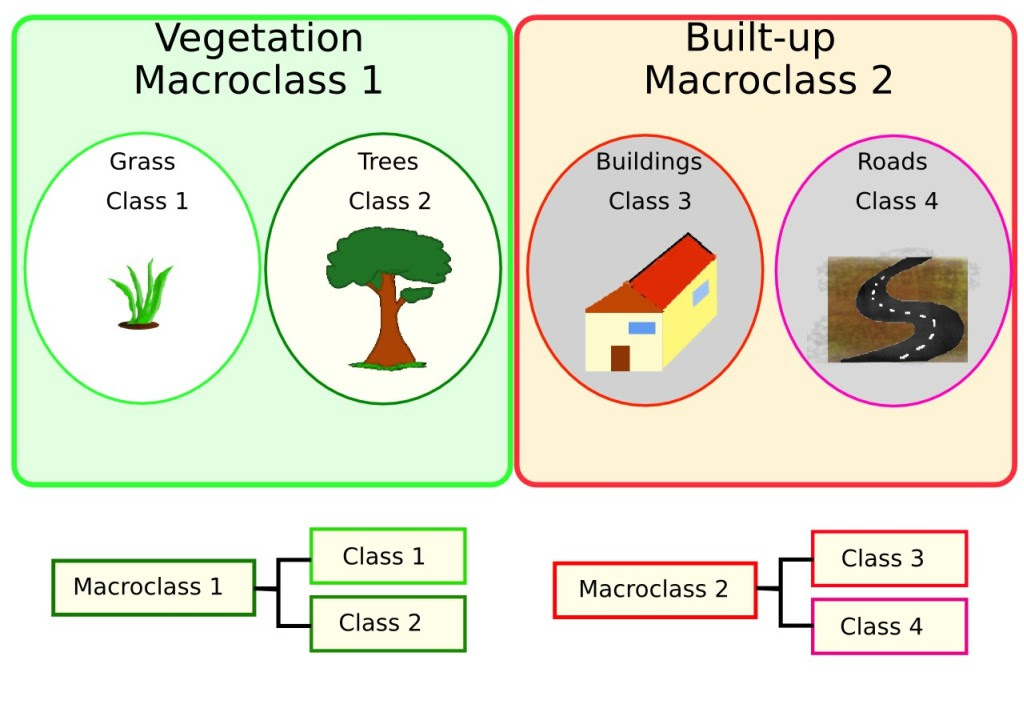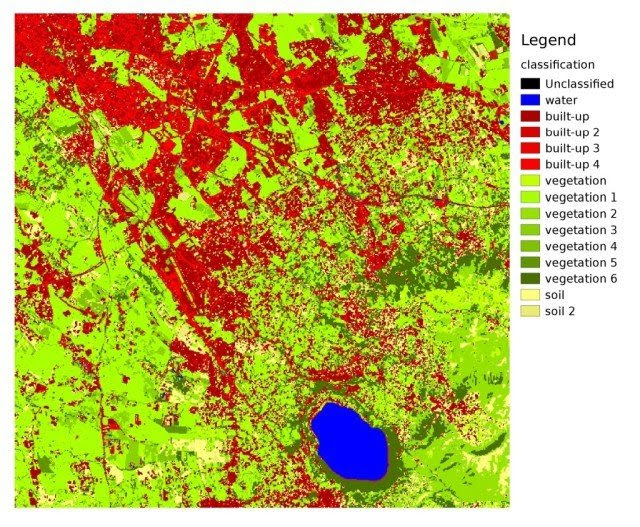### Brief Introduction to Remote Sensing (2/3): Supervised Classification Definitions

This is the second part of some basic definitions about remote sensing that are already in the user manual of the Semi-Automatic Classification Plugin.
This post provides basic definitions about supervised classifications.

### Land Cover

Land cover is the material at the ground, such as soil, vegetation, water, asphalt, etc. (Fisher and Unwin, 2005). Depending on the sensor resolutions, the number and kind of land cover classes that can be identified in the image can vary significantly.

### Supervised Classification

semi-automatic classification (also supervised classification) is an image processing technique that allows for the identification of materials in an image, according to their spectral signatures. There are several kinds of classification algorithms, but the general purpose is to produce a thematic map of the land cover.
Image processing and GIS spatial analyses require specific software such as the Semi-Automatic Classification Plugin for QGIS.

### Training Areas

Usually, supervised classifications require the user to select one or more Regions of Interest (ROIs, also Training Areas) for each land cover class identified in the image. ROIs are polygons drawn over homogeneous areas of the image that overlay pixels belonging to the same land cover class.

### Classes and Macroclasses

Land cover classes are identified with an arbitrary ID code (i.e. Identifier). SCP allows for the definition of Macroclass ID (i.e. MC ID) and Class ID (i.e. C ID), which are the identification codes of land cover classes. A Macroclass is a group of ROIs having different Class ID, which is useful when one needs to classify materials that have different spectral signatures in the same land cover class. For instance, one can identify grass (e.g. ID class = 1 and Macroclass ID = 1 ) and trees (e.g. ID class = 2 and Macroclass ID = 1 ) as vegetation class (e.g. Macroclass ID = 1 ). Multiple Class IDs can be assigned to the same Macroclass ID, but the same Class ID cannot be assigned to multiple Macroclass IDs, as shown in the following table.

Macroclass nameMacroclass IDClass nameClass ID
Vegetation1Grass1
Vegetation1Trees2

Therefore, Classes are subsets of a Macroclass as illustrated in Figure Macroclass example.Macroclass example

If the use of Macroclass is not required for the study purpose, then the same Macroclass ID can be defined for all the ROIs (e.g. Macroclass ID = 1) and Macroclass values are ignored in the classification process.

### Classification Algorithms

The spectral signatures (spectral characteristics) of reference land cover classes are calculated considering the values of pixels under each ROI having the same Class ID (or Macroclass ID). Therefore, the classification algorithm classifies the whole image by comparing the spectral characteristics of each pixel to the spectral characteristics of reference land cover classes. SCP implements the following classification algorithms.

#### Minimum Distance

Minimum Distance algorithm calculates the Euclidean distance d(x,y) between spectral signatures of image pixels and training spectral signatures, according to the following equation:

where:
• x = spectral signature vector of an image pixel;
• y = spectral signature vector of a training area;
• n = number of image bands.
Therefore, the distance is calculated for every pixel in the image, assigning the class of the spectral signature that is closer, according to the following discriminant function (adapted from Richards and Jia, 2006):

where:
• Ck = land cover class k;
• yk = spectral signature of class k;
• yj = spectral signature of class j.
It is possible to define a threshold Ti in order to exclude pixels below this value from the classification:

#### Maximum Likelihood

Maximum Likelihood algorithm calculates the probability distributions for the classes, related to Bayes’ theorem, estimating if a pixel belongs to a land cover class. In particular, the probability distributions for the classes are assumed the of form of multivariate normal models (Richards & Jia, 2006). In order to use this algorithm, a sufficient number of pixels is required for each training area allowing for the calculation of the covariance matrix. The discriminant function, described by Richards and Jia (2006), is calculated for every pixel as:

where:
• Ck = land cover class k;
• x = spectral signature vector of a image pixel;
• p(Ck) = probability that the correct class is Ck;
• |Σk| = determinant of the covariance matrix of the data in class Ck;
• Σ1k = inverse of the covariance matrix;
• yk = spectral signature vector of class k.
Therefore:

In addition, it is possible to define a threshold to the discriminant function in order to exclude pixels below this value from the classification. Considering a threshold Ti the classification condition becomes:

Maximum likelihood is one of the most common supervised classifications, however the classification process can be slower than Minimum Distance.

#### Spectra Angle Mapping

The Spectral Angle Mapping calculates the spectral angle between spectral signatures of image pixels and training spectral signatures. The spectral angle θ is defined as (Kruse et al., 1993):

Where:
• x = spectral signature vector of an image pixel;
• y = spectral signature vector of a training area;
• n = number of image bands.
Therefore a pixel belongs to the class having the lowest angle, that is:

where:
• Ck = land cover class k;
• yk = spectral signature of class k;
• yj = spectral signature of class j.
In order to exclude pixels below this value from the classification it is possible to define a threshold Ti:

Spectral Angle Mapping is largely used, especially with hyperspectral data.

### Spectral Distance

It is useful to evaluate the spectral distance (or separability) between training signatures or pixels, in order to assess if different classes that are too similar could cause classification errors. The SCP implements the following algorithms for assessing similarity of spectral signatures.

#### Jeffries-Matusita Distance

Jeffries-Matusita Distance calculates the separability of a pair of probability distributions. This can be particularly meaningful for evaluating the results of Maximum Likelihoodclassifications.
The Jeffries-Matusita Distance Jxy is calculated as (Richards and Jia, 2006):

where:

where:
• x = first spectral signature vector;
• y = second spectral signature vector;
• Σx = covariance matrix of sample x;
• Σy = covariance matrix of sample y;
The Jeffries-Matusita Distance is asymptotic to 2 when signatures are completely different, and tends to 0 when signatures are identical.

#### Spectral Angle

The Spectral Angle is the most appropriate for assessing the Spectra Angle Mapping algorithm. The spectral angle θ is defined as (Kruse et al., 1993):

Where:
• x = spectral signature vector of an image pixel;
• y = spectral signature vector of a training area;
• n = number of image bands.
Spectral angle goes from 0 when signatures are identical to 90 when signatures are completely different.

#### Euclidean Distance

The Euclidean Distance is particularly useful for the evaluating the result of Minimum Distance classifications. In fact, the distance is defined as:

where:
• x = first spectral signature vector;
• y = second spectral signature vector;
• n = number of image bands.
The Euclidean Distance is 0 when signatures are identical and tends to increase according to the spectral distance of signatures.

#### Bray-Curtis Similarity

The Bray-Curtis Similarity is a statistic used for assessing the relationship between two samples (read this). It is useful in general for assessing the similarity of spectral signatures, and Bray-Curtis Similarity S(x,y) is calculated as:

where:
• x = first spectral signature vector;
• y = second spectral signature vector;
• n = number of image bands.
The Bray-Curtis similarity is calculated as percentage and ranges from 0 when signatures are completely different to 100 when spectral signatures are identical.

### Classification Result

The result of the classification process is a raster (see an example of Landsat classification in Figure Landsat classification), where pixel values correspond to class IDs and each color represent a land cover class.Landsat classification
Data available from the U.S. Geological Survey
A certain amount of errors can occur in the land cover classification (i.e. pixels assigned to a wrong land cover class), due to spectral similarity of classes, or wrong class definition during the ROI collection.

### Accuracy Assessment

After the classification process, it is useful to assess the accuracy of land cover classification, in order to identify and measure map errors. Usually, accuracy assessment is performed with the calculation of an error matrix, which is a table that compares map information with reference data (i.e. ground truth data) for a number of sample areas (Congalton and Green, 2009).
The following table is a scheme of error matrix, where k is the number of classes identified in the land cover classification, and n is the total number of collected sample units. The items in the major diagonal (aii) are the number of samples correctly identified, while the other items are classification error.

Ground truth 1Ground truth 2Ground truth kTotal
Class 1a11a12a1ka1+
Class 2a21a22a2ka2+
Class kak1ak2akkak+
Totala+1a+2a+kn

Therefore, it is possible to calculate the overall accuracy as the ratio between the number of samples that are correctly classified (the sum of the major diagonal), and the total number of sample units n (Congalton and Green, 2009).
For further information, the following documentation is freely available: Landsat 7 Science Data User’s HandbookRemote Sensing Note , or Wikipedia.

References
• Congalton, R. and Green, K., 2009. Assessing the Accuracy of Remotely Sensed Data: Principles and Practices. Boca Raton, FL: CRC Press.
• Fisher, P. F. and Unwin, D. J., eds. 2005. Representing GIS. Chichester, England: John Wiley & Sons.
• JARS, 1993. Remote Sensing Note. Japan Association on Remote Sensing. Available at http://www.jars1974.net/pdf/rsnote_e.html
• Kruse, F. A., et al., 1993. The Spectral Image Processing System (SIPS) - Interactive Visualization and Analysis of Imaging spectrometer. Data Remote Sensing of Environment.
• NASA, 2013. Landsat 7 Science Data User’s Handbook. Available at http://landsathandbook.gsfc.nasa.gov
• Richards, J. A. and Jia, X., 2006. Remote Sensing Digital Image Analysis: An Introduction. Berlin, Germany: Springer.